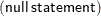## Syntax: OPTMODEL Procedure

PROC OPTMODEL statements are divided into three categories: the PROC statement, the declaration statements, and the programming statements. The PROC statement invokes the procedure and sets initial option values. The declaration statements declare optimization model components. The programming statements read and write data, invoke the solver, and print results. In the following text, the statements are listed in the order in which they are grouped, with declaration statements first.

Note: Solver specific options are described in the individual chapters that correspond to the solvers.

``` PROC OPTMODEL ``` options ;
``` CONSTRAINT ``` constraints ;
``` IMPVAR ``` optimization expression declarations ;
``` MAX ``` objective ;
``` MIN ``` objective ;
``` NUMBER ``` parameter declarations ;
``` PROBLEM ``` problem declaration ;
``` SET ```< types >parameter declarations ;
``` STRING ``` parameter declarations ;
``` VAR ``` variable declarations ;
``` Assignment ``` parameter = expression ;
``` CALL ``` name( expressions );
``` CLOSEFILE ``` files ;
``` CONTINUE ``` ;
``` CREATE DATA ``` SAS-data-set FROM columns ;
``` DO ``` ; statements ; END ;
``` DO ``` variable = specifications ; statements ; END ;
``` DO UNTIL ``` ( logic ) ; statements ; END ;
``` DO WHILE ``` ( logic ) ; statements ; END ;
``` DROP ``` constraint ;
``` EXPAND ``` name/ options;
``` FILE ``` file ;
``` FIX ``` variable= expression;
``` FOR ``` { index-set } statement ;
``` IF ``` logic THEN statement ;ELSE statement;
``` LEAVE ``` ;
`````` ;
``` PERFORMANCE ``` options ;
``` PRINT ``` print items ;
``` PUT ``` put items ;
``` QUIT ``` ;
``` READ DATA ``` SAS-data-set INTO columns ;
``` RESET OPTIONS ``` options ;
``` RESTORE ``` constraint ;
``` SAVE MPS ``` SAS-data-set( OBJECTIVE  |  OBJ ) name;
``` SAVE QPS ``` SAS-data-set( OBJECTIVE  |  OBJ ) name;
``` SOLVE ```WITH solverOBJECTIVE nameRELAXINT/ options;
``` STOP ``` ;
``` SUBMIT ``` arguments/ options;
``` UNFIX ``` variable= expression;
``` USE PROBLEM ``` problem ;